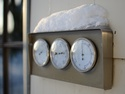## Rational Numbers

Type of Unit: Concept

# Prior Knowledge

Students should be able to:

• Solve problems with positive rational numbers.
• Plot positive rational numbers on a number line.
• Understand the equal sign.
• Use the greater than and less than symbols with positive numbers (not variables) and understand their relative positions on a number line.
• Recognize the first quadrant of the coordinate plane.

# Lesson Flow

The first part of this unit builds on the prerequisite skills needed to develop the concept of negative numbers, the opposites of numbers, and absolute value. The unit starts with a real-world application that uses negative numbers so that students understand the need for them. The unit then introduces the idea of the opposite of a number and its absolute value and compares the difference in the definitions. The number line and positions of numbers on the number line is at the heart of the unit, including comparing positions with less than or greater than symbols.

The second part of the unit deals with the coordinate plane and extends student knowledge to all four quadrants. Students graph geometric figures on the coordinate plane and do initial calculations of distances that are a straight line. Students conclude the unit by investigating the reflections of figures across the x- and y-axes on the coordinate plane.

Subject:
Mathematics, Numbers and Operations
Material Type:
Unit of Study
Level:
Middle School
6
Tags:
Integers, Absolute Value, Comparing Numbers, Statements, Number Line, Word Problems, Height, Inequality, 6th Grade Mathematics, Numbers, Problem Solving, Absolute Values, Graphing, Negative Numbers, Coordinate Plane, Graphing Points
Unit 1 Rational Numbers•••••Lesson 5

Inequalities
•••••Lesson 10

Reflections
••